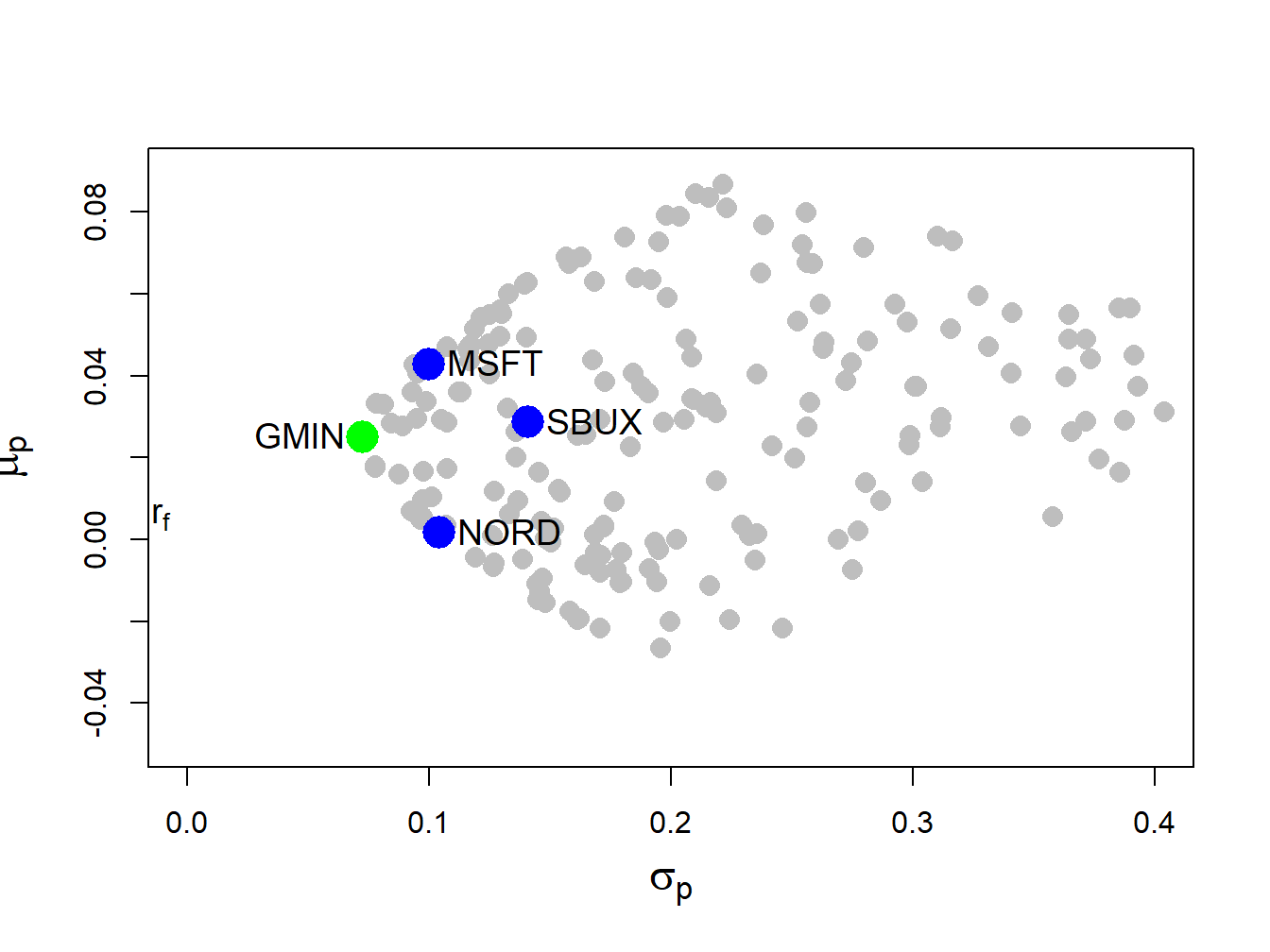## 12.2 Determining the Global Minimum Variance Portfolio Using Matrix Algebra

The global minimum variance portfolio $$\mathbf{m}=(m_{1},\ldots,m_{N})^{\prime}$$ for the $$N$$ asset case solves the constrained minimization problem: \begin{align} \min_{\mathbf{m}}~\sigma_{p,m}^{2}=\mathbf{m^{\prime}\Sigma m}\,s.t.\,\mathbf{m^{\prime}1}=1.\tag{12.2} \end{align} The Lagrangian for this problem is: \begin{align*} L(\mathbf{m},\lambda) & =\mathbf{m^{\prime}\Sigma m}+\lambda(\mathbf{m^{\prime}1}-1), \end{align*} and the first order conditions (FOCs) for a minimum are: \begin{align} \mathbf{0} & =\frac{\partial L}{\partial\mathbf{m}}=\frac{\partial\mathbf{m^{\prime}\Sigma m}}{\partial\mathbf{m}}+\frac{\partial\lambda(\mathbf{m^{\prime}1}-1)}{\partial\mathbf{m}}=2\Sigma \mathbf{m}+\lambda\mathbf{1},\tag{12.3}\\ 0 & =\frac{\partial L}{\partial\lambda}=\frac{\partial\mathbf{m^{\prime}\Sigma m}}{\partial\lambda}+\frac{\partial\lambda(\mathbf{m^{\prime}1}-1)}{\partial\lambda}=\mathbf{m^{\prime}1}-1.\tag{12.4} \end{align} The FOCs (12.3)-(12.4) give $$N+1$$ linear equations in $$N+1$$ unknowns which can be solved to find the global minimum variance portfolio weight vector $$\mathbf{m}$$ as follows. The $$N+1$$ linear equations describing the first order conditions have the matrix representation: $\begin{equation} \left(\begin{array}{cc} 2\Sigma & \mathbf{1}\\ \mathbf{1}^{\prime} & 0 \end{array}\right)\left(\begin{array}{c} \mathbf{m}\\ \lambda \end{array}\right)=\left(\begin{array}{c} \mathbf{0}\\ 1 \end{array}\right).\tag{12.5} \end{equation}$ The system (12.5) is of the form $$\mathbf{A}_{m}\mathbf{z}_{m}=\mathbf{b}$$, where: $\mathbf{A}_{m}=\left(\begin{array}{cc} 2\Sigma & \mathbf{1}\\ \mathbf{1}^{\prime} & 0 \end{array}\right),~\mathbf{z}_{m}=\left(\begin{array}{c} \mathbf{m}\\ \lambda \end{array}\right)\textrm{ and }\mathbf{b}=\left(\begin{array}{c} \mathbf{0}\\ 1 \end{array}\right).$ Provided $$\mathbf{A}_{m}$$ is invertible, the solution for $$\mathbf{z}_{m}$$ is:79 $\begin{equation} \mathbf{z}_{m}=\mathbf{A}_{m}^{-1}\mathbf{b}.\tag{12.6} \end{equation}$ The first $$N$$ elements of $$\mathbf{z}_{m}$$ is the portfolio weight vector $$\mathbf{m}$$ for the global minimum variance portfolio with expected return $$\mu_{p,m}=\mathbf{m}^{\prime}\mu$$ and variance $$\sigma_{p,m}^{2}=\mathbf{m}^{\prime}\Sigma \mathbf{m}$$.

Example 2.6 (Global minimum variance portfolio for example data)

Using the data in Table 12.1, we can use R to compute the global minimum variance portfolio weights from (12.6) as follows:

top.mat = cbind(2*sigma.mat, rep(1, 3))
bot.vec = c(rep(1, 3), 0)
Am.mat = rbind(top.mat, bot.vec)
b.vec = c(rep(0, 3), 1)
z.m.mat = solve(Am.mat)%*%b.vec
m.vec = z.m.mat[1:3,1]
m.vec
##  MSFT  NORD  SBUX
## 0.441 0.366 0.193

Hence, the global minimum variance portfolio has portfolio weights $$m_{\textrm{msft}}=0.441$$, $$m_{\textrm{nord}}=0.366$$ and $$m_{\textrm{sbux}}=0.193$$, and is given by the vector $$\mathbf{m}=(0.441,0.366,0.193)^{\prime}$$. The expected return on this portfolio, $$\mu_{p,m}=\mathbf{m}^{\prime}\mu$$, is:

mu.gmin = as.numeric(crossprod(m.vec, mu.vec))
mu.gmin
##  0.0249

The portfolio variance, $$\sigma_{p,m}^{2}=\mathbf{m}^{\prime}\Sigma \mathbf{m}$$, and standard deviation, $$\sigma_{p,m}$$, are:

sig2.gmin = as.numeric(t(m.vec)%*%sigma.mat%*%m.vec)
sig.gmin = sqrt(sig2.gmin)
c(sig2.gmin, sig.gmin)
##  0.00528 0.07268

In Figure 12.4, this portfolio is labeled “GMIN” .Figure 12.4: Global minimum variance portfolio from example data.

$$\blacksquare$$

### 12.2.1 Alternative derivation of global minimum variance portfolio

We can use the first order conditions (12.3) - (12.4) to give an explicit solution for the global minimum variance portfolio $$\mathbf{m}$$ as follows. First, use (12.3) to solve for $$\mathbf{m}$$ as a function of $$\lambda$$: $\begin{equation} \mathbf{m}=-\frac{1}{2}\cdot\lambda\Sigma^{-1}\mathbf{1}.\tag{12.7} \end{equation}$ Next, multiply both sides of (12.7) by $$\mathbf{1}^{\prime}$$ and use (12.4) to solve for $$\lambda$$: \begin{align*} 1 & =\mathbf{1}^{\prime}\mathbf{m}=-\frac{1}{2}\cdot\lambda\mathbf{1}^{\prime}\Sigma^{-1}\mathbf{1}\\ & \Rightarrow\lambda=-2\cdot\frac{1}{\mathbf{1}^{\prime}\Sigma^{-1}\mathbf{1}}. \end{align*} Finally, substitute the value for $$\lambda$$ back into (12.7) to solve for $$\mathbf{m}$$: $\begin{equation} \mathbf{m}=-\frac{1}{2}\left(-2\right)\frac{1}{\mathbf{1}^{\prime}\Sigma^{-1}\mathbf{1}}\Sigma^{-1}\mathbf{1}=\frac{\Sigma^{-1}\mathbf{1}}{\mathbf{1}^{\prime}\Sigma^{-1}\mathbf{1}}.\tag{12.8} \end{equation}$ Notice that (12.8) shows that a solution for $$\mathbf{m}$$ exists as long as $$\Sigma$$ is invertible.

Example 2.9 (Finding global minimum variance portfolio for example data)

Using the data in Table 12.1, we can use R to compute the global minimum variance portfolio weights from (12.8) as follows:

one.vec = rep(1, 3)
sigma.inv.mat = solve(sigma.mat)
top.mat = sigma.inv.mat%*%one.vec
bot.val = as.numeric((t(one.vec)%*%sigma.inv.mat%*%one.vec))
m.mat = top.mat/bot.val
m.mat[,1]
##  MSFT  NORD  SBUX
## 0.441 0.366 0.193

$$\blacksquare$$

1. For $$\mathbf{A}_{m}$$ to be invertible we need $$\Sigma$$ to be invertible, which requires $$|\rho_{ij}|\neq1$$ for $$i\neq j$$ and $$\sigma_{i}^{2}>0$$ for all $$i$$.↩︎